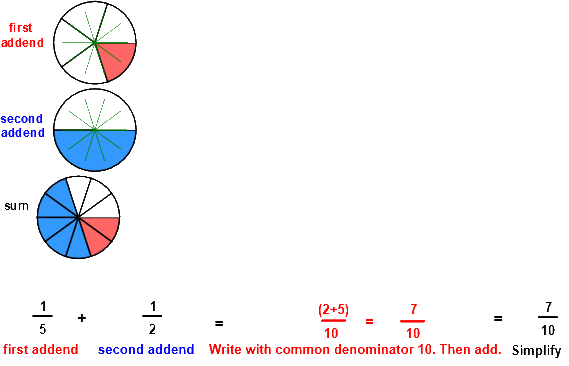CORRECT:
ATTEMPTS:
SCORE:
PERCENT
NUMERATOR
DENOMINATOR

# ADDITION OF UNLIKE FRACTIONS INSTRUCTION<

Follow the directions in the dialog box after pressing the <START> button. The <EXPLAIN> button may be pressed after you enter the second addend to see how to do the exampleNotice that the addends 15 and 12 have unlike denominators. You must first rename 15 and 12 with like or common denominators(LCD).  The common denominator for 5 and 2 is 10 because both 5 and 2 divide evenly into 10.

The program COMPARE FRACTIONS shows how to find the LCD.

The picture shows that each addend is divided into tenths. So the first addend 15 can be written as 210 as shown in red. The second addend 12 can be written as 510 as shown in blue.

Now you can add the first and second addends because 210 and 510 have like denominators. This is shown in the sum image where the 210 and 510 are combined in red and blue to give the sum 710.

Once each addend is written with like denominators the numerators may be added for the sum numerator.

For more instruction on adding fractions go to How To Add Fractions.

After you enter the sum you may press the <REPORT> button. The report will ask for your name but you may submit a code for your name. This report will give the same results as on the dialog box. The report may be printed or e-mailed.